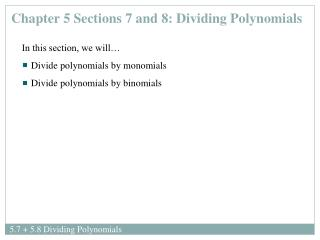DownloadDownload PresentationChapter 5 Sections 7 and 8: Dividing Polynomials

# Chapter 5 Sections 7 and 8: Dividing Polynomials

Download Presentation## Chapter 5 Sections 7 and 8: Dividing Polynomials

- - - - - - - - - - - - - - - - - - - - - - - - - - - E N D - - - - - - - - - - - - - - - - - - - - - - - - - - -
##### Presentation Transcript

1. Chapter 5Sections 7 and 8: Dividing Polynomials • In this section, we will… • Divide polynomials by monomials • Divide polynomials by binomials

2. Dividing Polynomials more than one term is in the denominator - this will require long-division of polynomials. one term in the denominator -simply divide each term in the numerator by the denominator. Examples:Will the following require long-division? Explain.

3. To divide polynomials – use the Order of Operations and the Laws of Exponents Examples:Perform the division.

4. Examples:Perform the division.

5. Examples:Perform the division.

6. Examples:Perform the division.

7. Example: Divide using long division with integers. answer: Is 5 a factor of 82? Explain. check: quotient divisor dividend To check your result: (quotient) (divisor) + remainder = dividend

8. Example: Find the quotient and the remainder when and check your result. • Write the division in long-division form • Ensure that each polynomial is in descending order • Insert a placeholder for missing terms (e.g. leave a space, 0x)

9. Example:Find the quotient and the remainder when and check your result. check

10. Example: Find the quotient and the remainder when and check your result. check

11. Example: Find the quotient and the remainder when and check your result. check

12. Independent Practice You learn math by doing math. The best way to learn math is to practice, practice, practice. The assigned homework examples provide you with an opportunity to practice. Be sure to complete every assigned problem (or more if you need additional practice). Check your answers to the odd-numbered problems in the back of the text to see whether you have correctly solved each problem; rework all problems that are incorrect. Read pp. 442-445 and pp.448-451 Homework: pp. 446-448 #2, 3, 4, 17-23 odds, 29-47 odds, 55, 69, 71, 75, 83-88 all pp. 452-454 #2, 5, 7, 9, 37-43 odds, 51, 55, 59